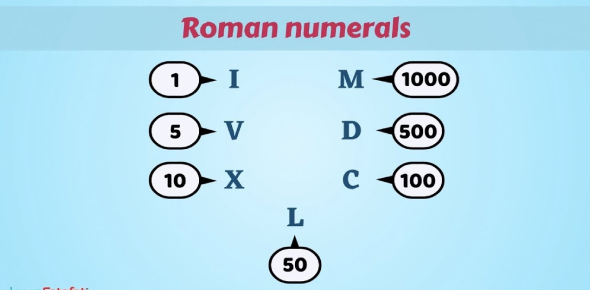# Math Test On Roman Numerals

13 Questions | Total Attempts: 18239SettingsThe numeric system represented by Roman numerals originated in ancient Rome. See how well you do own Roman numerals and can you correctly change it back to normal numbers? I hope you enjoy the quiz that tests out the answer you just gave! Give it a try!

Related Topics
• 1.
• A.

45

• B.

38

• C.

789

• 2.
What number is this XLVII?
• A.

89

• B.

47

• 3.
What number is this XCI?
• A.

91

• B.

200

• 4.
What number is this XLII?
• A.

42

• B.

92

• 5.
What number is this LXXI?
• A.

81

• B.

71

• 6.
What number is this XCII?
• A.

92

• B.

258

• C.

32

• 7.
What number is this XCIII?
• A.

93

• B.

89

• C.

75

• 8.
What number is this LV?
• A.

14

• B.

55

• C.

23

• 9.
What number is this XVI?
• A.

16

• B.

65

• C.

12300

• 10.
What number is this LXV?
• A.

89

• B.

32

• C.

65

• 11.
What number is this XLI?
• A.

6565

• B.

12

• C.

41

• 12.
What number is this LXXXIII?
• A.

28798

• B.

83

• C.

63

• 13.
What number is this XLVI?
• A.

11

• B.

1289

• C.

46